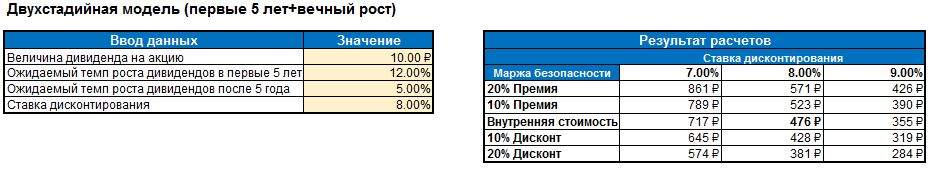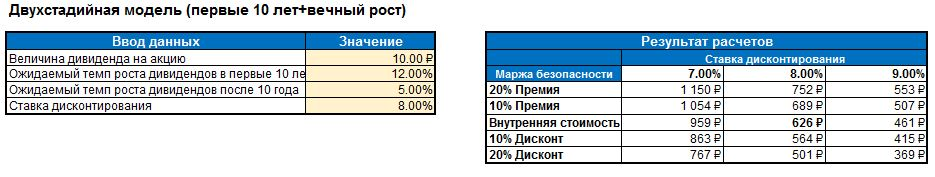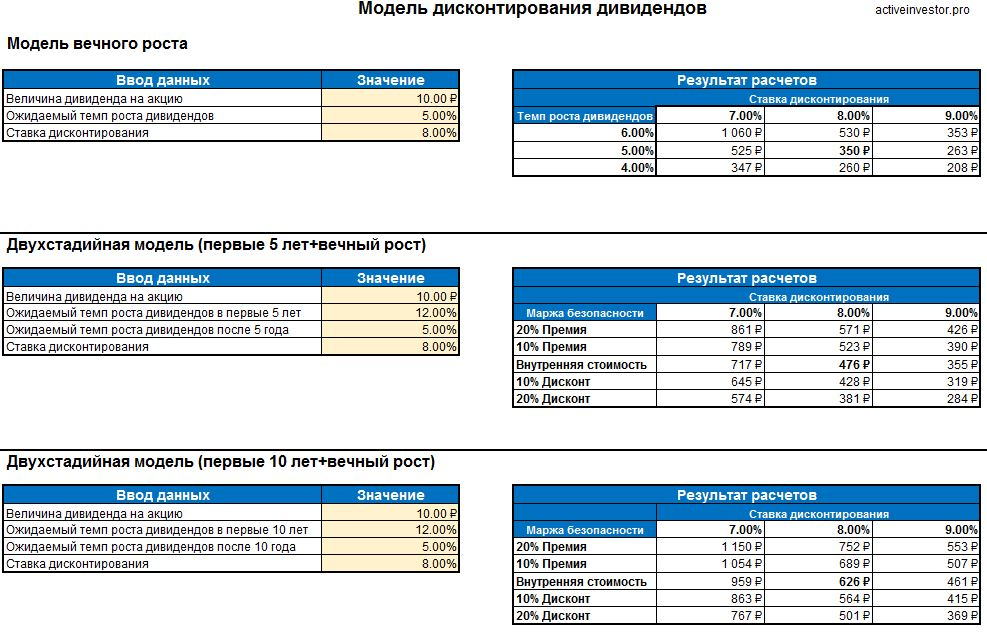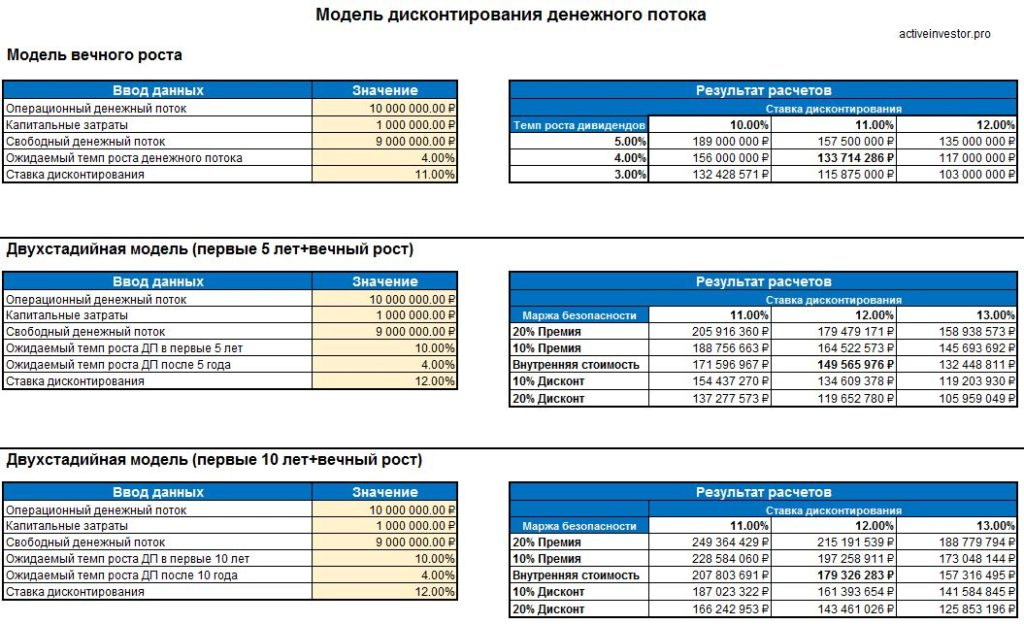Forex News

# Stock Valuation CalculatorOne of the basic rules of an active investor who buys shares is not to pay more than the asset is worth. But for this, you first need to determine how much this asset is worth.

Discounting cash flows is one of the main ways to determine the fair value of shares. But it involves a lot of calculations, especially when it comes to complex models. In this article, I will briefly recall how this model works using the example of dividends and show a calculator with which you can quickly calculate the fair value of a share.## How does dividend discounting work?

Dividend discounting is a version of the cash flow discounting model designed to measure dividend-paying stocks. The essence of the model is reduced to a simple principle:

The value of the investment today is equal to the sum of all future cash flows that will be received, with each of these cash flows discounted to their present value.

Routine discounted cash flow analysis estimates the company’s future cash flow potential and uses these estimated cash flows to determine the company’s fair value today.

The dividend discounting model uses the potential dividends that the shares will pay in the future as cash flows. Therefore, the model sums up all estimated future dividends and then discounts them to their present value. Thus, the share price is equal to the total of all future discounted dividends.

DCF = D1 / (1 + r) + D2 / (1 + r) ^ 2 +… + Dn / (1 + r) ^ n

• DCF – the sum of all future discounted cash flows (or dividends per share, in this case). In other words, this is the fair value we are looking for.
• D– dividends per share paid in a given year. D1 for the first year, D2 for the second year, and so on.
• ris the discount rate in decimal form. This is the rate of return that you want to receive from your investment.

If we simplify this model, the formula will look like this:

DCF = D1 / (rg)

• D1 is the projected dividend per share for next year.
• r is your desired rate of return
• g is the assumed long-term average annual growth rate of dividends.

## How to use the dividend discounting model?

I’ll show you how this model works with an example. Let’s say a company plans to pay 10 rubles per share this year. And you want to get an 8% return on your investment. Plus you expect the company to increase its dividend by an average of 5% per year. In this case, the fair price of the share is: 10 * (1 + 0.05) / (0.08-0.05) = 350 rubles.

We should not forget about the principle of safety margin. It is not a fact that in reality dividends and growth rates will turn out to be the same as you predicted. Therefore, you should buy a stock with a safety margin – for example, for 300 rubles.

The main disadvantage of this model is that it is very sensitive to changes. Suppose, in our example, dividends would grow not by 5%, but by 4%. In this case, we get a completely different cost – 260 rubles. And if dividends grow by 6%, then the cost will be 530 rubles. This gives us a very wide range of fair values. Therefore, this model is suitable for companies that have stable and predictable dividend growth rates.

But there is a way out of this situation – a two-stage discounting model. In the early stages of their development, companies can maintain high growth rates in their profits and dividends. But sooner or later, any company will face a ceiling and its growth rate will decline. The two-stage model allows for one early dividend growth rate and another mature dividend growth rate. The calculation formula in this case becomes more complicated, so it is much easier to use a special calculator to evaluate stocks.

## How to estimate the value of a share?

In order not to do these calculations manually, you can use a special calculator for evaluating stocks made in Excel. I use this stock calculator to analyze the companies I review on my second blog. Here is an example calculation where the current dividend of 10 rubles is used, the growth rate in the first 5 years is 12%, the growth rate after 5 years is 5% and the rate of return is 8%.

As a result of calculations, the cost is 476 rubles. Also, the calculator immediately calculates and shows the cost in case of a different discount rate and taking into account the security margin of 10% and 20%.Sometimes high growth rates of profits and dividends can last for more than 5 years. In this case, the calculator will calculate the growth rate in the first 10 years and the growth rate after 10 years. In this case, the price is 626 rubles.When using a calculator, it is important not to overestimate the growth rate. This is especially true for the second stage, where growth rates are projected virtually “forever”. These growth rates cannot exceed the average growth rate of the economy.

## How do I use the calculator?

The calculator is an Excel file. It consists of two sheets: DDM – sheet for calculating using the dividend discounting model, DCF – for calculating using the discounted cash flow method. The calculator has the ability to make an assessment using three models:

1. Perpetual growth model – dividends grow at the same rate of growth forever.
2. Model for the first 5 years + eternal growth. This is a two-stage model, where the first 5 years dividends grow at one rate of growth, after 5 years and indefinitely – at a different rate.
3. Model for the first 10 years + eternal growth. This is a two-stage model, where for the first 10 years dividends grow at one rate of growth, after 10 years and indefinitely at a different rate.

To calculate, you need to enter several parameters in the yellow cells: the current amount of the dividend, the expected growth rate and the discount rate (your required rate of return).

The calculator will automatically calculate and display the result in the table next to the right. The table will contain the fair value based on the inputs. Also, the calculator will immediately calculate the value based on similar parameters of the discount rate and the growth rate of dividends – you do not have to enter them separately and you will immediately get a range of the fair value of shares.The second DCF sheet is intended for valuation by discounting free cash flow. In this case, as a result, you get not the value of a share, but the value of the entire company – its capitalization. In principle, this method is similar to how the dividend discounting model works. For the calculation, you must enter the amount of operating cash flow and the amount of capital costs. The calculator will calculate the free cash flow itself. If you are using a different cash flow formula, you can enter your calculated amount in this field.### How do I get the Stock Valuation Calculator?

You can get the calculator by making a payment using the form below. The calculator in the form of an xlsx file will be sent to the e-mail specified during ordering within 24 hours.

Share on social networks

### Polak Donovan

Hello! My name is Polak Donovan, I am a private investor, I have been trading currencies in Forex since 2011, I have increased my capital by 70% with growing dynamics. During this time, I have accumulated a lot of experience that I would like to share in my blog. I will tell you how to speculate in the market and at the same time reduce the beginner's mistakes. I will show my results and share my predictions, I also collected the most relevant news for you on the site, which, in my opinion, affect market trends.